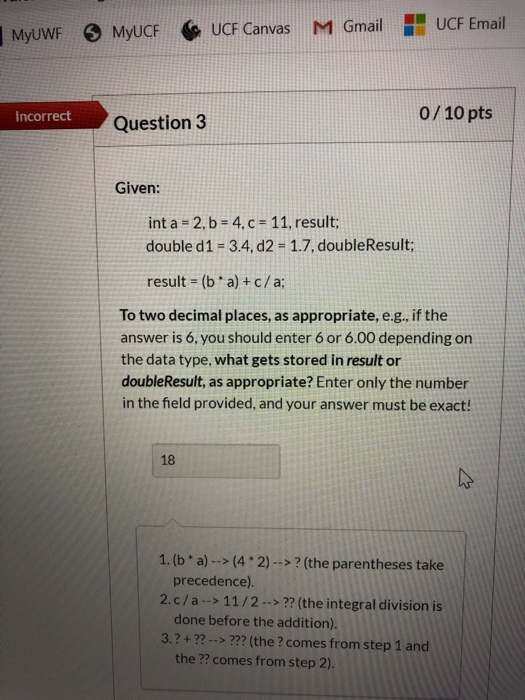Home / Expert Answers / Other / myuwf-myucfc-ucf-canvas-ucf-email-m-gmail-incorrect-question-3-0-10-pts-given-int-a-2-b-4-c-11-resul

# (Solved): MyUWF MYUCFC UCF Canvas UCF Email M Gmail Incorrect Question 3 0/10 pts Given: int a = 2, b = 4, C ...MyUWF MYUCFC UCF Canvas UCF Email M Gmail Incorrect Question 3 0/10 pts Given: int a = 2, b = 4, C = 11, result; double d1 = 3.4, d2 = 1.7, doubleResult; result = ( ba) + c/a: To two decimal places, as appropriate, e.g., if the answer is 6, you should enter 6 or 6.00 depending on the data type, what gets stored in result or doubleResult, as appropriate? Enter only the number in the field provided, and your answer must be exact! 1.(ba) --> (4.2) -->? (the parentheses take precedence). 2.c/a --> 11/2 --> ?? (the integral division is done before the addition). 3.?+ ?? --> ??? (the ? comes from step 1 and the ?? comes from step 2).

We have an Answer from Expert